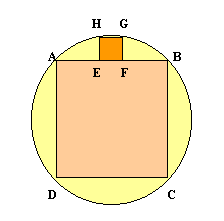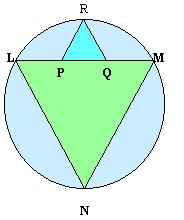#### You may also like### Doodles

Draw a 'doodle' - a closed intersecting curve drawn without taking pencil from paper. What can you prove about the intersections?### Russian Cubes

I want some cubes painted with three blue faces and three red faces. How many different cubes can be painted like that?### Picture Story

Can you see how this picture illustrates the formula for the sum of the first six cube numbers?

# Fitting In

##### Age 14 to 16Challenge Level

The largest square which fits into a circle is $ABCD$ and $EFGH$ is a square with $E$ and $F$ on the line $AB$ and $G$ and $H$ on the circumference of the circle. Show that $AB = 5EF$.Similarly the largest equilateral triangle which fits into a circle is $LMN$ and $PQR$ is an equilateral triangle with $P$ and $Q$ on the line $LM$ and $R$ on the circumference of the circle. Show that $LM = 3PQ$.